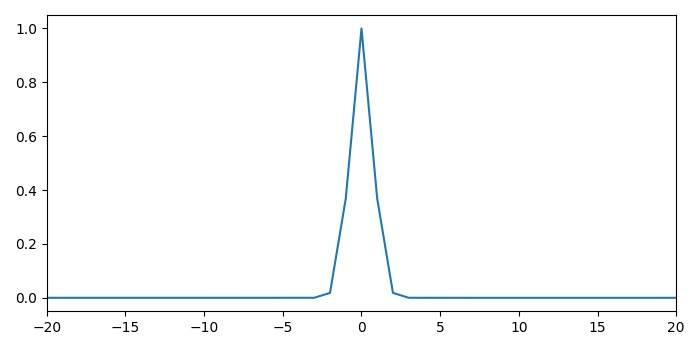# Plotting a probability density function by sample with Matplotlib

To plot a probability density function by sample, we can use numpy for x and y data points.

## Steps

• Create x and p data points using numpy.
• Plot x and p data points using plot() method.
• Scale X-axis in a range.
• To display the figure, use show() method.

## Example

import numpy as np
from matplotlib import pyplot as plt
plt.rcParams["figure.figsize"] = [7.00, 3.50]
plt.rcParams["figure.autolayout"] = True
x = np.arange(-100, 100)
p = np.exp(-x ** 2)
plt.plot(x, p)
plt.xlim(-20, 20)
plt.show()

## Output